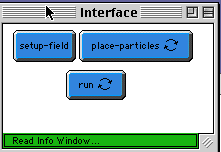WHAT IS IT?
-----------

This is a mathematical model that demonstrates abstract vector fields and
integral curves.  The vector field chosen for this particular model is

- y d/dx  +  x d/dy

The integral curves are trajectories of particles which move in the vector
fields.  In this case all trajectories should be concentric circles.  However, in
this model each particle moves one space before calculating its new heading, so
it doesn't turn quite as much as if its heading were recalculated continuously.
Therefore the integral curves will spiral outward.

HOW TO USE IT
-------------

1) Click on the SETUP-FIELDS button.  SETUP-FIELDS will clear the screen and
compute the vector field.  The field is plotted using vector graphics: green
streaks are individual vectors with yellow turtles serving as arrow heads.  The
length of each vector is roughly proportional to the magnitude of the the vector
field at each point.  In this case it is just the distance from the origin.

2) Click the PLACE-PARTICLES button.  This forever button puts the program into
the mode in which you can position red test-particles by clicking anywhere in the
graphics window.

3) To see the integral curves, click on RUN.

EXTENDING THE MODEL
-------------------

Try a different vector field.  Change it in the "setup-fields" procedure.    For
instance, if you choose

x d/dx - y d/dy

the integral curves will be hyperbolas.

; This model was created as part of the project: CONNECTED MATHEMATICS:
; MAKING SENSE OF COMPLEX PHENOMENA THROUGH BUILDING OBJECT-BASED PARALLEL
; MODELS (OBPML)
; The project gratefully acknowledges the support of the National Science
; Foundation (Applications of Advanced Technologies Program) -- grant numbers
; RED #9552950 and REC #9632612.
;